## some typesetting solutions

All definitions are designed to be used within math mode unless otherwise specified.
updated September 19, 2009.

logic
description
definition
command
output
non-monotonic squiggle/snake \newcommand{\nc}{\,\mid\!\sim\,} \nc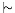non-monotonic consequence \newcommand{\nm}{\,\mid\!\approx\,} \nm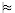non-decomposable entailment \newcommand{\entailsM}{\,\mid\!\bumpeq\,} \entailsM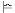modal satisfaction \newcommand{\vm}{
$\Vdash^{\mathcal{M}}_{#1_{{#2}}}$}
\vm{w}{1}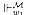\vm{w}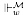\vm{}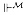necessity operator \newcommand{\nec}{\nabla} \nec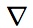possibility operator \newcommand{\pos}{
\rotatebox[origin=c]{180}{$\nabla$}}
\pos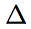contraction operator \newcommand{\dotminus}{
\buildrel\textstyle.\over{\hbox{
\vrule height3pt depth0pt width0pt}{\smash-}
}}
\dotminus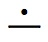probability

description
definition
command
output
credal set \def\crs{\mathbb{K}} \crs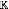c-dagger \newcommand{\cdagger}{\bm{\mathrm c^\dagger}} \cdagger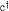c-star \newcommand{\cstar}{\bm{\mathrm c^*}} \cstar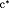evidential certainties \def\Gd{\Gamma_{\delta}} \Gd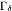independence* \newcommand\ind{
\protect\mathpalette{\protect\independenT}{\perp}}
\def\independenT#1#2{
\mathrel{\rlap{$#1#2$}\mkern2mu{#1#2}}}
\ind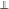* thanks to Jon Williamson

sets and lists
description
definition
command
output
set construction \newcommand{\set}{
\left\{#1\colon#2\right\}}
\set{f(x)}{x\in A}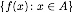n-tuples, in text mode or math mode; sets variables to san serif à la Barwise & Etchemendy* \newcommand{\ntuple}{
{\sf #1}$_{1}$,\ldots,{\sf #1}$_{#2}$}
\ntuple{\phi}{n}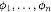\ntuple{P}{n}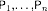* thanks to Anthony Dardis

beamer
description
code

When <arg> is empty, all navigation symbols are removed. For other options, see Matthias Pospiech's page.

Getting natbib to work with beamer*

Insert \def\newblock{} immediately before your \bibliography{<arg>}.

* thanks to Jonah Schupbach